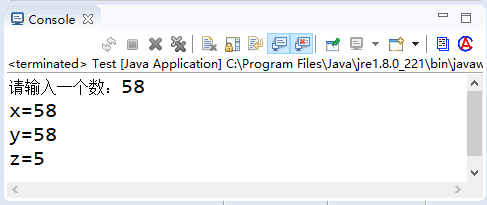# java三元运算符的值如何确定？java三元运算符怎么使用？`判断条件? 表达式1 :表达式2`

java三元运算符怎么使用?

```int x, y, z;
x = 6, y = 2;
z = x > y ? x - y : x + y;```

`x>y ? x-=y : x+=y;`

`(x>y ? x-=y : x)+=y;`

`(x>y) ? (x-=y): (x+=y);`

```public class Test9
{
public static void main(String[] args)
{
int x, y, z; // 声明三个变量
System.out.print("请输入一个数：");
Scanner input = new Scanner(System.in);
x = input.nextInt(); // 由用户输入x的值
// 判断x的值是否大于5，如果是y=x，否则y=-x
y = x > 5 ? x : -x;
// 判断y的值是否大于x，如果是z=y，否则z=5
z = y > x ? y : 5;
System.out.printf("x=%d \n", x);
System.out.printf("y=%d \n", y);
System.out.printf("z=%d \n", z);
}
}```qt怎么运行别人的项目?qt运行项目流程